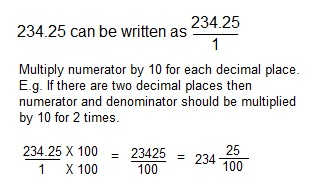## Decimal-Fraction conversion

Decimal system is a commonly used number system where every number is expressed in base ten. Each place has a value which is obtained by multiplying 10 as many times as the position of the number minus 1

E.g. 234=2 X 10X10 + 2 X 10 + 4 X 0

If we go from right to left place becomes 10 times smaller.

Consider the number 234.25. "." is called the decimal point. As we move from right to left after decimal point place becomes 10 times smaller.

234.25: 234 is the whole part and .25 is the decimal part.

234.25 can be written as 200 + 30 + 4 + 2/10 + 5/100. This is called the expanded form of the decimal.

Conversion of decimal to fraction

1. Write the number in the form of a fraction with 1 as the denominator.
2. Mutliply numerator and denominator by as many 10 as there are decimal places.

E.g.